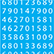Online Program

Saturday, June 1The SAMSI Program on Model Uncertainty
Sat, Jun 1, 1:00 PM - 2:35 PM
Grand Ballroom I

The Stochastic Inverse Problem (305046)

Troy Butler, University of Colorado Denver
Donald Estep, Colorado State University
*Lei Yang, SAMSI

Keywords: Probability distribution, Quantities of Interest, Stochastic Inverse Problem

Mathematical models of physical systems, often in the form of differential equations, are common in many scientific and engineering disciplines. Such models induce a map between the parameter domain and the range determined by Quantities of Interest(QoI) computed from the model. Usually, such map is not invertible, so its inverse maps into a space of equivalence classes in the parameter domain that generalizes the notion of a contour map, rather than the parameter domain itself.

The Stochastic Inverse Problem (SIP) is defined as the problem of calculating a probability distribution on the parameter domain which induces a given probability distribution on the range. Our research is primarily concerned with the formulation and numerical algorithm for the SIP. When the Uniform Ansatz is employed, the solution probability density has a unique explicit formula. Furthermore, under proper condition of the domain boundary, we prove this solution density is continuous. The continuity result is a sufficient condition for the convergence of the proposed numerical method for the SIP. The second part of our work concerns the extension of the formulation and solution of the SIP to the case where the domain is a cube of countable dimension. We show that the solution of the infinite dimensional SIP exists, and can be approximated by the solution of a finite dimensional SIP with continuity property.